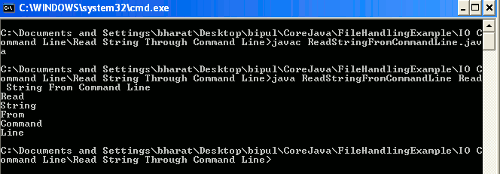Tutorials

# How To Read String From Command Line In Java

In Java command line argument is entered when an application is invoking. This command line argument is entered after giving the name of the class. There is no limit of arguments giving from the command line

In Java command line argument is entered when an application is invoking. This command line argument is entered after giving the name of the class. There is no limit of arguments giving from the command line

## How To Read String From Command Line In Java

In this section we will discuss about how string can be read through the command line.

In Java command line argument is entered when an application is invoking. This command line argument is entered after giving the name of the class. There is no limit of arguments giving from the command line. Command line arguments are passed to the application's main method through the array of strings.

Example

This example demonstrates that how to take input from command line. Here in this example we will give the input as command line using the keyboard. Here I am giving the two Java source code, one will explain that how any number of arguments can be read given as command line and the other will explain that how we a specified number of argument can be read given as argument.

1. In first type of example I have created a Java class named ReadStringFromCommandLine.java where I have used while loop to read any number of input from the command line. In while loop I have compared an integer variable with the length of command line argument in such a way that if the value of integer variable is less than the length of argument till then print the value of arguments given from the command line.

Source Code

```public class ReadStringFromCommandLine
{
public static void main (String[] args)
{
int i =0;
while(i < args.length)
{
String s = args[i];
System.out.println(s);
i++;
}
}
}
```

2. In second type of example I have created a Java class named ReadStringFromCommandLine1.java where I have checked a condition that whether the length of argument is greater than zero or not i.e. whether the argument, after the class name, is given or not when invoking the class.

Source Code

```public class ReadStringFromCommandLine1
{
public static void main(String args[])
{
if (args.length > 0)
{
try{
String s = args;
System.out.println("First argument = "+s);
String s1 = args;
System.out.println("Second argument = "+s1);
}
catch (ArrayIndexOutOfBoundsException e)
{
System.err.println(e);
}
}
}
}
```

Output

When you will execute the source code ReadStringFromCommandLine.java then the output will be as follows :When you will execute the source code ReadStringFromCommandLine1.java then the output will be as follows :Source code of above both examples can be downloaded from the link given below. In the downloaded file you will get the two Java files ReadStringFromCommandLine.java and ReadStringFromCommandLine1.java.